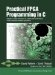# Section 6.4. Using HDL Simulation to Understand Stream Protocols

### 6.4. Using HDL Simulation to Understand Stream Protocols

The easiest way to understand the generated stream interfaces and their communication protocols is to examine them in the context of a simulation test bench. Figures 6-7 presents such a test bench for the FIR filter example. When used in a VHDL simulator, this test bench applies the coefficient and waveform data from the same files used in the software simulation of Chapter 5.

The important parts of this VHDL test bench are the two loops that apply the data from the input files to the stream. One of these loops (the one that applies the actual waveform data) appears as follows:

` k := 0; while true loop     p_producer_process_waveform_raw_en <= '0';     if (p_producer_process_waveform_raw_rdy = '1') then         p_producer_process_waveform_raw_data <= testval_table(k);         p_producer_process_waveform_raw_en <= '1';         k := k+1;     end if;     wait until rising_edge(clk);     if k=testval_count then         exit;     end if; end loop; `

This loop defines the following basic protocol, which applies to all stream inputs:

1. Set the _en control line low (value '0'). This tells the stream that the data currently appearing on the _data input is no longer valid.

2. Wait for the _rdy line (which is an output status signal from the stream) to be asserted. This can be done by using a VHDL wait statement sensitive to the _rdy input, or by checking the value of _rdy at a clock edge, as just shown above.

3. When _rdy is asserted, assign the desired data value to the _data input of the stream.

4. Set the _en line high (value '1'). This instructs the stream to accept the data. The stream will accept that data at the next rising clock edge.

5. After the clock edge, set the _en low (step 1).

To accept data from an output stream the steps are similar, with the _en signal being used to indicate to the stream that a value has been successfully read and the stream may be updated with the next value. In the FIR filter test bench, the _en control signal is simply held high, allowing values to be observed directly during the course of the simulation.

##### Figure 6-7. VHDL test bench for the FIR filter.
` -- FIR filter test bench -- library ieee; use ieee.std_logic_1164.all; use ieee.std_logic_arith.all; use ieee.std_logic_textio.all; use ieee.std_logic_arith.all; use std.textio.all; use work.config.all; entity test_top is end test_top; architecture behavior of test_top is  signal reset, clk : std_ulogic;  signal p_producer_process_waveform_raw_en : std_ulogic;  signal p_producer_process_waveform_raw_eos : std_ulogic;  signal p_producer_process_waveform_raw_data :                                         std_ulogic_vector (31 downto 0);  signal p_producer_process_waveform_raw_rdy : std_ulogic;  signal p_consumer_process_waveform_filtered_en : std_ulogic;  signal p_consumer_process_waveform_filtered_data :                                          std_ulogic_vector (31 downto 0);  signal p_consumer_process_waveform_filtered_eos : std_ulogic;  signal p_consumer_process_waveform_filtered_rdy : std_ulogic;  constant PERIOD: time := 10 ns; component fir_arch is  port (   reset, sclk, clk : in std_ulogic;   p_producer_process_waveform_raw_en : in std_ulogic;   p_producer_process_waveform_raw_eos : in std_ulogic;   p_producer_process_waveform_raw_data :                                     in std_ulogic_vector (31 downto 0);   p_producer_process_waveform_raw_rdy : out std_ulogic;   p_consumer_process_waveform_filtered_en : in std_ulogic;   p_consumer_process_waveform_filtered_data :                                     out std_ulogic_vector (31 downto 0);   p_consumer_process_waveform_filtered_eos : out std_ulogic;   p_consumer_process_waveform_filtered_rdy : out std_ulogic); end component fir_arch; begin   reset_stimulus: process   begin    reset <= '1'; wait for PERIOD;    reset <= '0'; wait;   end process;   clk_stimulus: process   begin     clk <= '0'; wait for PERIOD/2;     clk <= '1'; wait for PERIOD/2;   end process;   stimulus: process    variable k : integer;    begin     p_producer_process_waveform_raw_eos <= '0';     p_producer_process_waveform_raw_en <= '0';     p_producer_process_waveform_raw_data <= X"00000000";     wait for PERIOD;     -- First output the coefficients     k := 0;     while true loop       p_producer_process_waveform_raw_en <= '0';       if (p_producer_process_waveform_raw_rdy = '1') then           p_producer_process_waveform_raw_data <= coef_table(k);           p_producer_process_waveform_raw_en <= '1';           k := k+1;       end if;       wait until rising_edge(clk);       if k=coef_count then           exit;       end if;     end loop;     -- Now output the waveform values     k := 0;     while true loop       p_producer_process_waveform_raw_en <= '0';       if (p_producer_process_waveform_raw_rdy = '1') then           p_producer_process_waveform_raw_data <= testval_table(k);           p_producer_process_waveform_raw_en <= '1';           k := k+1;         end if;         wait until rising_edge(clk);       if k=testval_count then         exit;       end if;     end loop;   end process stimulus;   results: process   begin     p_consumer_process_waveform_filtered_en <= '1';     -- Now compare to the results of the software encryption     while (p_consumer_process_waveform_filtered_eos = '0') loop       -- results will appear on p_consumer_process_waveform_raw_data       wait until rising_edge(clk);     end loop;     wait;   end process;    -- Instantiate the design under test    DUT: entity work.fir_arch     port map (       reset, sclk, clk,       p_producer_process_waveform_raw_en,       p_producer_process_waveform_raw_eos,       p_producer_process_waveform_raw_data,       p_producer_process_waveform_raw_rdy,       p_consumer_process_waveform_filtered_en,       p_consumer_process_waveform_filtered_data,       p_consumer_process_waveform_filtered_eos,       p_consumer_process_waveform_filtered_rdy     ); end behavior; `Practical FPGA Programming in C
ISBN: 0131543180
EAN: 2147483647
Year: 2005
Pages: 208